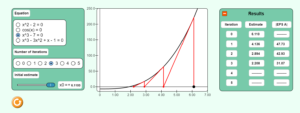# Chapter 03 Newton Raphson Method Simulation

Description:  This simulation depicts the Newton-Raphson Method of solving nonlinear equations.  The student can explore the way the root estimate converges or diverges, and also note the rate of convergence.  They can also simulate the pitfalls of Newton- Raphson method in action such as near division by zero, and root jumping.Keywords:  roots of nonlinear equations, Newton Raphson method.

Learning Objectives:  After successful completion of running the simulation with proper questions asked by the instructor, the student would be able to 1) note the convergence and divergence of the method 2) the open nature of the method, 3) rate of convergence, 4) study the pitfalls of the method such as root jumping, near division by zero.

Full Resources:  The full resources for the topic of the Newton-Raphson method are given here which include textbook content, a PowerPoint presentation, a multiple-choice test, audiovisual lectures, and application examples.